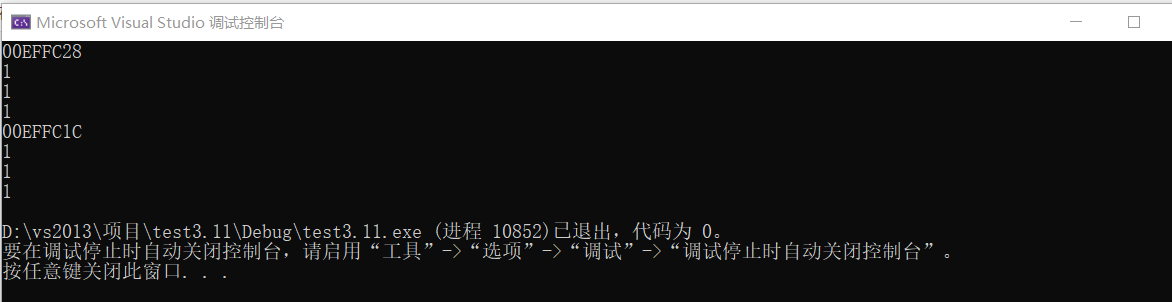## this

this指针的类型取决于使用this 的成员函数类型以及对象类型，this指针并不是成员的一部分，sizeof无用

``````#include<iostream>
using namespace std;
class A
{
public:
A()
{
m =1;
}
void Display()
{
cout << this << endl;
cout << (*this).m << endl;
cout << this->m << endl;
cout << m << endl;
}
private:
int m;
};
int main()
{
A a;
A b;
a.Display();
b.Display();
}
``````struct默认为public，class默认为private